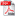# EFFICIENT COMPUTATIONS WITH STRUCTURED MATRIX PENCILS IN AUTOMATIC CONTROL

Autor/autori: Vasile SIMA

Abstract: There is a continuous research effort worldwide to improve the reliability, efficiency, and accuracy of numerical calculations in various domains. One of the most promising research avenue is to exploit the structural properties of the mathematical problems to be solved. This paper investigates some improved algorithms for the solution of skew-Hamiltonian/Hamiltonian eigenproblems, which have applications not only in automatic control (linear-quadratic optimization and H∞-optimization), but also for multi-body systems, and in various areas of applied mathematics, physics, and chemistry. Such eigenproblems involve matrix pencils in which one matrix is skew-Hamiltonian and the other is either Hamiltonian or skew-Hamiltonian. Of much interest is to find the eigenvalues and certain deflating subspaces, mainly those associated to the stable eigenvalues. So-called J-congruence transformations are used, which preserve the pencil structure. By employing unitary J-congruence transformations, the best accuracy of the solutions is guaranteed, since the problem numerical conditioning is preserved. The algorithms reduce the original problem, or an equivalent problem of double size, to a structured Schur form, which reveals the eigenvalues. The embedding is needed when the structured Schur form does not exist for the original problem. The pencil matrices may have either real or complex entries

Keywords: Deflating subspaces, eigenvalue reordering, generalized eigenvalues, generalized Schur form, numerical algorithms, skew-Hamiltonian/Hamiltonian matrix pencil, software, structure-preservationDOWNLOAD PDF